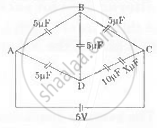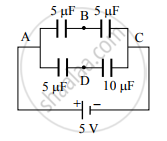Share

# Six capacitors of capacities 5, 5. 5, 5, 10 and X μ F are connected as shown in the network in the diagram - Physics

#### Question

Six capacitors of capacities 5, 5. 5, 5, 10 and X μ F are connected as shown in the network in the diagram.

Find :

1. The value of X if the network is balanced, and
2. The resultant capacitance between A and C.#### Solution

Given balanced network of capacitors with
CAB = CBC = CAD = CBD = 5 µF, CDC = (10 + X) µF
The value of X = ?
Resultant capacitance between A and C (Ceq) = ?

a.Using formula, C_s=(C_1C_2)/(C_1+C_2) for branch DC of the given network we get

C_s=(10xxX)/(10+X)    ..........(i)

Now using formula

C_(AB)/C_(BC)=C_(AD)/C_(DC) (For balance condition)

∴ 5/5=5/((10xxX)/(10+X))

∴ 1=(10+X)/(2X)

∴ 2X=10+X

∴ x=10muF

The value of X is 10 µF.

b. As the bridge is balanced, no current flows through branch BD. Hence the network can be reduced as follows:Here, 5 µF and 5 µF are in series in the branch ABC.
Using formula (i),

C_s=(5xx5)/(5+5)=2.5muF

Also, 5 µF and 10 µF are in series in the branch ADC.

C_s^('')=(5xx10)/(10+10)=50/20=2.5 muF

Now , C_s^(') and C_s^('') are in parallel

∴ CAC = CP = CS′ + CS′′ = 2.5 µF + 2.5 µF
= 5 µF

∴ The resultant capacitance between A and C is 5 µF.

Is there an error in this question or solution?